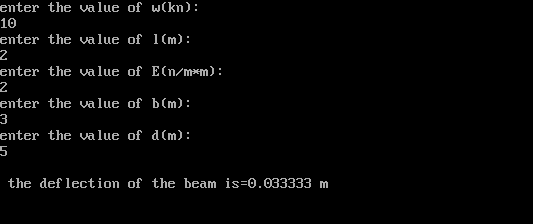### C program for determination of deflection of UDL on simply supported beam

/*program for determination of deflection of UDL on simply supported beam*/

#include<stdio.h>
#include<math.h>
void main()
{
float w,l,E,y,i,b,d;
/*w=weight,l-length,E=modulus of elasticity*/
/*i=moment of inertia,y=deflection,b=span,d=depth*/
clrscr();
printf("enter the value of w(kn):\n");
scanf("%f",&w);
printf("enter the value of l(m):\n");
scanf("%f",&l);
printf("enter the value of E(n/m*m):\n");
scanf("%f",&E);
printf("enter the value of b(m):\n");
scanf("%f",&b);
printf("enter the value of d(m):\n");
scanf("%f",&d);
i=(b*pow(d,3)/12.0);
/*calculation of deflection of beam*/
y=(5.0/384.0)*(w*pow(l,4)/(E*i));
printf("\n the deflection of the beam is=%f m",y);
getch();
}
/*OUTPUT*/Show us your appreciation by liking or sharing this post:
Previous
Next Post »